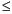Functions and CALL Routines

# RANTRI Function

Returns a random variate from a triangular distribution.
 Category: Random Number Tip: If you want to change the seed value during execution, you must use the CALL RANTRI routine instead of the RANTRI function.

## Syntax

 RANTRI(seed,h)

### Arguments

seed

is a numeric constant, variable, or expression with an integer value. If seed0, the time of day is used to initialize the seed stream.

h

is a numeric constant, variable, or expression that specifies the mode of the distribution.

 range: 0 < h < 1

The RANTRI function returns a variate that is generated from the triangular distribution on the interval (0,1) with parameter h, which is the modal value of the distribution. An inverse transform method applied to a RANUNI uniform variate is used.

A triangular distribution X on the interval (A,B) with mode C, where ACB, can be generated:

`x=(b-a)*rantri(seed,(c-a)/(b-a))+a;`

For a discussion about seeds and streams of data, as well as examples of using the random-number functions, see Generating Multiple Variables from One Seed in Random-Number Functions.

The CALL RANTRI routine, an alternative to the RANTRI function, gives greater control of the seed and random number streams.

 Functions and CALL routines:Previous Page | Next Page | Top of Page